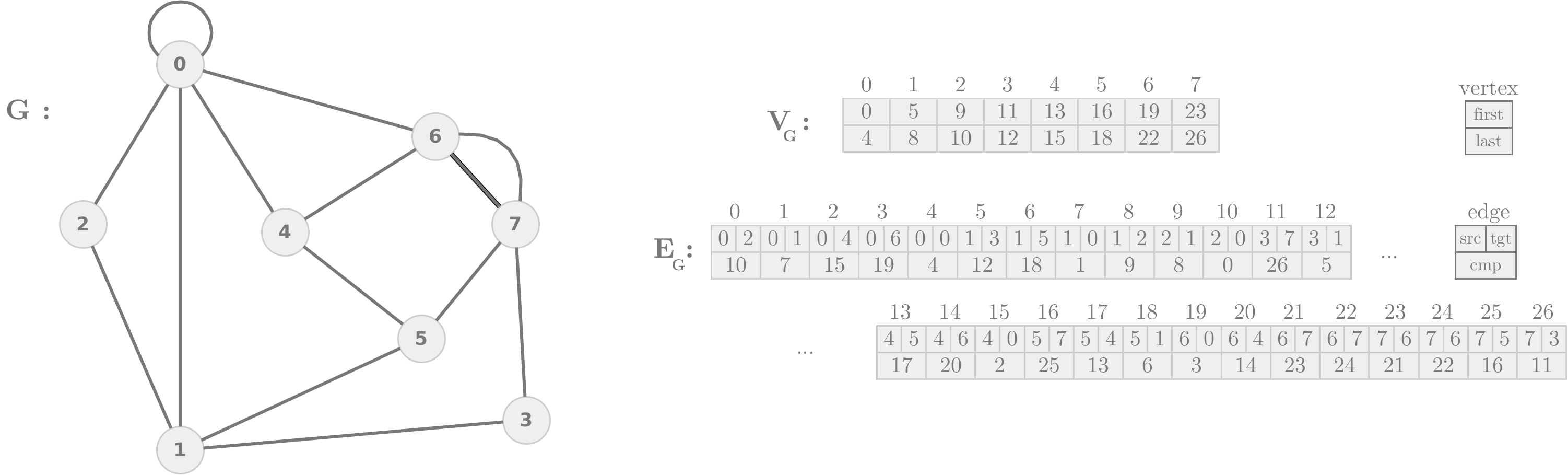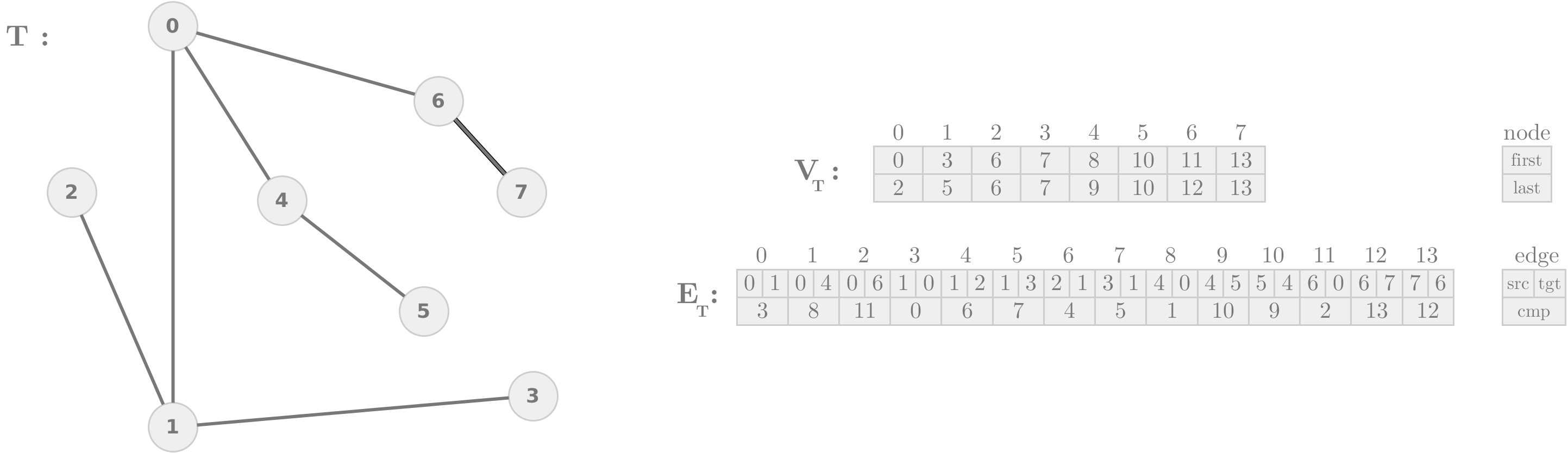# Read, write and create datasets

In this section we provide functions to read and write our datasets, and create new ones.

## Graphs

To navigate our graphs, we use the following representation:

Let G be a planar graph with a planar embedding. G is represented by an array of vertices, VG, and an array of edges, EG. Each vertex v ∈ V G stores two indices in EG, v.first and v.last, indicating the adjacency list of v, sorted counterclockwise around v. Notice that the number of neighbors of v is (v.last - v.first + 1). Each edge e ∈ EG has three fields, e.src, which is a pointer to the source vertex, e.tgt, which is a pointer to the target vertex and e.cmp, which is the position in EG of the complement edge of e, e', where the e'.src = e.tgt and e'.tgt = e.src. For example, see the following graph and its representation.To support multiple edges, the indices of the multiple edges must be always increasing. In other words, the adjacency list of a vertex cannot start in the middle of a list of multiple edges.

• Read and write graphs: Read and write graphs. This code convert a graph from our datasets to the representation above.
• Build planar graphs: Build a planar graph with a planar embedding. We provide a set of scripts in bash and python to generate planar graphs based on Delaunay triangulations. To use the scripts, the following softwares have to be installed: Python3 (package numpy), Triangle and The Edge Addition Planarity Suite.

## Trees

To navigate our trees, we use the same representation used in graphs. The only difference is that the adjacency list of a node starts with the edge to the parent of such node (except the root). Notice that the number of children of a node v is (v.last - v.first). For example, see the following tree and its representation.• Read and write trees: Read and write trees. This code convert a tree from our datasets to the representation above.
• Spanning tree of a graph: Compute a spanning tree of a graph, assuming the previous representations of graphs and tree.